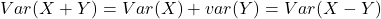# Expected Values or Mathematical Expectations

The expected value, or mean, of a random variable is a measure of the central location for the random variable. The formula for the expected value of a discrete random variable X follows.
Expected value of a discrete random variable X can be written as i.e.,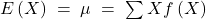Properties of Mathematical Expectations [Expected Values]

1. The expected value of constant ‘C’ is equal to the constant, .i.e.,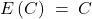2. The expected value of the product of a constant ‘C’ and a random variable X is equal to the constant times the expected values of random variable. i.e.,3. The expected value of the sum of a random variable X and a constant ‘C’ is the sum of the expected values of the random variable and constant. i.e.,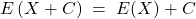4. The expected value of the product of two independent random variable is equal to the product of their individual expected value. i.e.,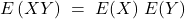5. The expected value of the sum of two independent random variable is equal to the sum of their individual expected values. i.e.,6. The variance of the product of a constant and a random variable X is equal to the constant squared times the variance of the random variable X. i.e.,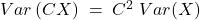7. The variance of the sum of two independent random variables is equal to the sum of their individual variances. Also, the variance of the difference of two independent random variables is equal to the sum of their individual variances. i.e.,